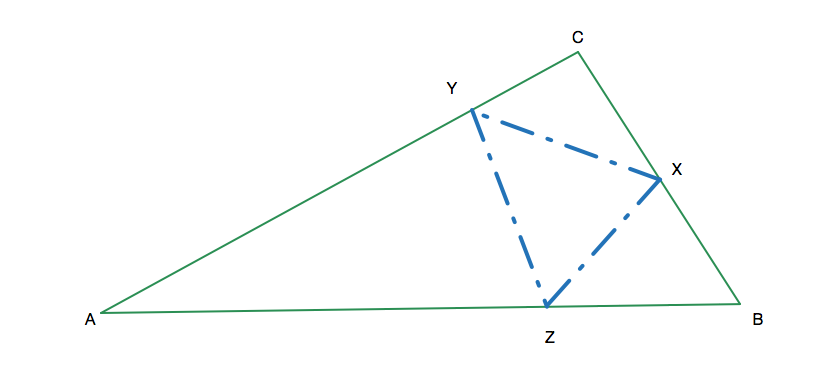Bouncing off of the Sides of A Triangle Forever

Geometry Level 5Let $ABC$ be a triangle such that $BC=5$, $CA=8$, and $AB=9$. A ball is launched from a point $X$ on segment $BC$ such that it first bounces off of segment $CA$ at a point $Y$, then bounces off of segment $AB$ at a point $Z$, then bounces off of segment $BC$ at $X$, then bounces off of segment $CA$ at $Y$, and so on, traversing the perimeter of triangle $XYZ$ forever.

The area of triangle $XYZ$ can be written in the form $\tfrac{p\sqrt{q}}{r}$, where $p$ and $r$ are coprime positive integers and $q$ is a squarefree integer. Find $p+q+r$.

×